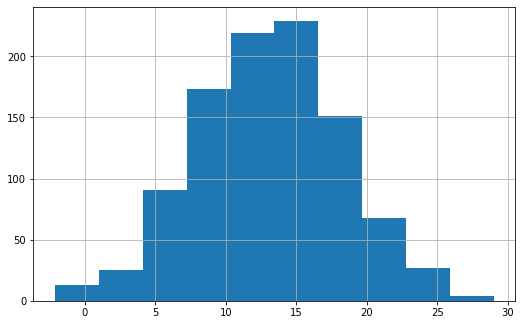How to manage image resolution of a graph in Matplotlib

Machine LearningServer Side ProgrammingProgrammingMatplotlib

An Image contains a 2-D matrix RGB data points which can be defined by the dots point per inch [ DPI ] of the image. The resolution of the image is important because a hi-resolution image will have much more clarity.

We have a method ‘plt.savefig()’ in Matplotlib which determines the size of the image in terms of its pixels. Ideally it is having an ‘dpi’ parameter.

Let’s see how we can manage the resolution of a graph in Matplotlib.

Example

import matplotlib.pyplot as plt
import numpy as np

#Prepare the data for histogram
np.random.seed(1961)
nd = np.random.normal(13, 5, 1000)

#Define the size of the plot
plt.figure(figsize=(8,6))
plt.hist(nd)
plt.grid()

#set the dpi value to 300
plt.savefig('histogram_img.png', dpi=300)
plt.show()
plt.figure(figsize=(18,12))
plt.hist(nd)
plt.grid()

#Set the dpi value to 150
plt.savefig('histogram_100.png', dpi=150)
plt.show()

Running the above code will generate the output as,

Output